## Place value in the millions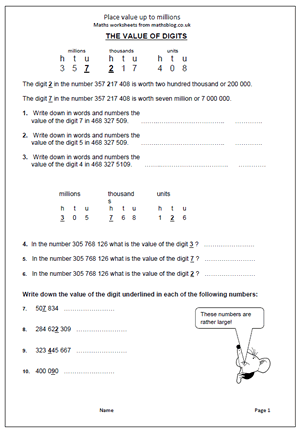Children are expected to work with even larger numbers in Year 5, including millions. Many will have difficulties in reading and writing such large numbers and will need a lot of help to appreciate the place value of each digit.

The easiest way to read  large numbers is to think of them as blocks of three, starting on the right.

Each block of three is hundreds, tens and units, which keep repeating themselves.

The first block of three are HUNDREDS, TENS AND UNITS of ones or units.

So  456 is four hundred and fifty six (units).

The second block of three are HUNDREDS, TENS AND UNITS of thousands.

So 456 456 is four hundred and fifty six thousand four hundred and fifty six.

This page takes a closer look at reading large numbers and finding the value of various digits.

Place value up to millions

## Resource of the Week: Year 5 Number Challenge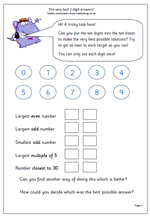I really like this challenge, partly because there is no, one right way to answer it and partly because it really makes children think.

There are 10 digits, from zero to nine to be placed in the 10 boxes in such a way that the targets can be matched as closely as possible. The catch is that each number can only be used once!

Now the obvious way to start is to make 98 the largest even number, but immediately that means that you can not have 97 as the largest odd number. By the time you reach the last target, number closest to 30, you have only two digits left and only two choices!

But what counts as the best possible answers? This is as big a challenge, if not bigger. I have had a class try to make a set of rules to try to be as fair as possible, but it involved a great deal of addition and subtraction. One group made a set of rules that went like this:

1. Find the difference between 98 and the answer given.

2. Find the difference between 99 and the answer given.

This will give your total so far – the larger the total, the worse you have done.

I won’t continue with this as it might spoil the fun!

This page can be found in our Year 5, Using and Understanding Maths category.

## Place value to millions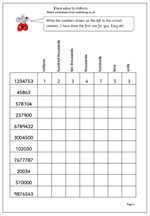Many children find reading large numbers very difficult, as indeed do many adults. This is a very simple page which looks at reading larger numbers up to millions. If nothing else it should help children realise that they need to start with hundreds tens and units and the the next three columns are then repeated but in thousands. A common error is to start trying to read a very large number from the largest digit when this can often just be a guess.

Working in blocks of three:

123 456 789

789 hundreds tens units

456 hundreds tens units of thousands

123 hundreds tens units of millions

so the number is 123 million 456 thousand and 789.

Easy!

Place value: millions

## Year 5 Knowing multiplication facts to help with division.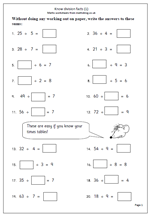There is no doubt that most children find division harder than multiplication, yet there is little real reason for this to be so. Most division questions can be turned on their head and knowledge of times tables used to work them out. For example:

30 ÷ 5 =??  can be thought as what number times 5 makes 30?

?? ÷ 6 = 7 can be thought of as 6 times 7.

60 ÷ ?? = 10 can be thought of as what number times 10 makes 60?

The key to success with division is to have a really good knowledge of times tables.

This worksheet looks at these types of division question, all with easy numbers and no remainders and is a good assessment sheet to see if division is understood and tables known. if times tables are not known sufficiently well children will spend more time than necessary trying to work these out.

This can be found in our Year 5 Knowing Number Facts category.

Know division facts (1)

## Scalene triangles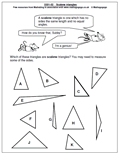A lot of work is done on triangles in Primary Schools and by year 5 and 6 most children are familiar with the properties of right angled triangles, equilateral triangles and isosceles trinagles. However, fewer children know about the scalene triangle. A scalene triangle is one which has no sides the same length and no equal angles. It is worth pointing out that right angled triangles could also be scalene if all the sides are different lengths.

Thanks to MathSphere Ltd for this worksheet: and there are many more similar pages, both on their site and part of the ‘It’s All Figured Out’ CD.

Go to our year 5 shape resources

## Resource of the Week: written addition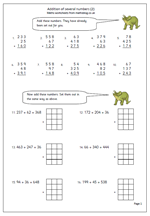Written methods of calculating continue to prove to be some of the more popular pages on the site. This week I would like to highlight a Year 5 maths worksheet on written addition which is the second in a series showing children clearly how addition should be laid out and also giving them the opportunity to re-write questions from a horizontal lay out to a vertical layout. The hardest part of this is to ensure the numbers line up under each other, starting with the units on the far right. Squares can help with this in the early stages, but children should also get used to laying them out correctly without squared paper.

Rarely, if ever, will you find a SATs addition question which has been laid out already in the traditional, or standard method but it is expected that children use this method when solving a difficult addition calculation.

This pager can be found in our Year 5 Calculating section. Similar pages, with more detailed instructions, can also be found in our Four Rules section.

Written addition of several numbers (2)

## Year 5 division problems: calculating mentally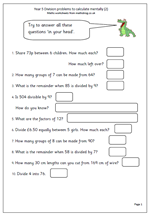Here is the second page which looks at Year 5 division problems that can be answered by using mental skills. The questions cover a range of concepts involving division, including:

remainders

divisibility rule for 9

and factors.

Watch out for errors that occur in questions such as number 9, where the number of whole lengths of wire is calculated and the remainder is irrelevant.

This page, and other similar worksheets can be found in the Year 5 calculating category.

Division problems (2)

## Understanding factors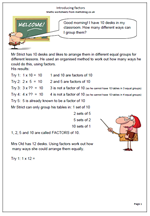Understanding factors can prove to be quite tricky, but in fact is a fairly simple concept, with once again knowing times tables being crucial. These pages begin by trying to find the factors of a number on an organised ‘trial and error’ basis by seeing which numbers when multiplied together will make the target number.

When doing this it saves time if it is understood when to stop.

For example; the factors of 10:

Try 1:  1 x 10 =  10        1 and 10 are factors of 10
Try 2:  2 x  5  =  10        2 and 5 are factors of 10
Try 3:  3 x ??  =  10       3 is not a factor of 10 (so he cannot have 10 tables in 3 equal groups)
Try 4:  4 x ??  =  10       4 is not a factor of 10 (so he cannot have 10 tables in 4 equal groups)
Try 5:  5 is already known to be a factor of 10 2 x 5 is the same as 5 x 2. There is no need to go further than this.

Introducing factors

## Resource of the Week: percentagesThis week’s resource of the Week highlights the second of our percentages worksheets for Year 5. It is typical of the English that we manage to write per cent in two different ways. Unlike the Americans who use percent we use two words per cent, apart from when we write percentage when we put it altogether.Anyway, as to the maths: remember that many percentages can be worked out ‘in your head’ by remembering that 10% of an amount is equivalent to dividing the amount by 10. At this stage we will only be dealing with whole tens so it is probably the best approach. Later more complicated percentages will be found by dividing by 100 and multiplying by the percentage.

To find 20% of £350.

Find 10% of £350, which  is £35

If 10% is £35, then 20% will be £35 x 2 = £70.

Percentages (pg 2)

## Year 5 maths worksheet: solve single step word problems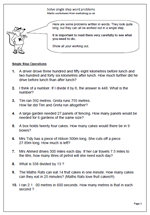This page contains a set of ten problems written in words. They look quite long, but they can all be worked out using a single step, using one of the operations: addition, subtraction, multiplication and division. Because they only require one step they are easier than questions which involve two or steps, but each calculation is quite tricky, with several needing a good knowledge of written methods to be able to do them.

It is important that all working out is shown for several reasons, including to show that a calculator was not used and to be able to spot where errors occur. Wrong answers are an excellent step towards new learning!

It is also interesting to see how children approach the questions. One or two questions in particular may cause problems. Question 6, involves subtracting of decimals and can be laid out in the standard format but could also be answered by adding on: e.g.

27.89 + 0.11 = 28

28 + 72 = 100

100 + 200 = 300

answer = 0.11 + 72 + 200 = 272.11

Solve single step word problems